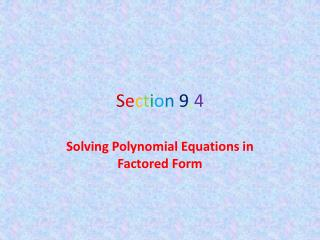# S e c t i o n 9 . 4 - PowerPoint PPT PresentationDownload PresentationS e c t i o n 9 . 4

S e c t i o n 9 . 4Download Presentation## S e c t i o n 9 . 4

- - - - - - - - - - - - - - - - - - - - - - - - - - - E N D - - - - - - - - - - - - - - - - - - - - - - - - - - -
##### Presentation Transcript

1. Section9.4 Solving Polynomial Equations in Factored Form

2. Algebra I Notes Section 9.4: Solving Polynomial Equations in Factored Form In this lesson we will learn how to solve quadratic and other polynomial equations in factored form. A polynomial is in ________________ if it is written as the product of two or more linear factors. When solving equations in factored form we must use the _____________________ to find the solutions. Zero Product Property If ab = 0, then a = 0 or b = 0 or both a & b = 0 Examples: Solve the polynomial equations using the zero product property. 1) (x – 4)(x + 1) = 0 2) factored form zero product property A binomial so nice, write it twice x – 4 = 0 x + 1 = 0 x + 8 = 0 x + 8 = 0 x = 4 x = -1 x = -8 (Dbl. Root)

3. 3) (3x – 2)(4x + 3)(x + 4) = 0 • Sometimes to solve a polynomial equation using the zero-product property, you may need to factor out the ________________________________ of the polynomial’s terms. This is a monomial with an integer coefficient that divides evenly into each term • or a common variable in every term. • Example: Factor out the greatest common monomial factor. • 4) 8x + 12y 5) 14y2 – 21y 3x – 2 = 0 4x + 3 = 0 x + 4 = 0 3x = 2 4x = -3 x = -4 x = 2/3 x = -3/4 greatest common monomial factor 4(2x + 3y) 7y(2y – 3)

4. Examples: Solve the following equations using GCF. • 6 ) 3x2+ 18x = 0 7) 4x2 = 14x 3x(x + 6) = 0 4x2 – 14x = 0 2x(2x – 7) = 0 3x = 0 x + 6 = 0 2x = 0 2x – 7 = 0 x = 0 x = -6 x = 0 2x = 7 x = 7/2